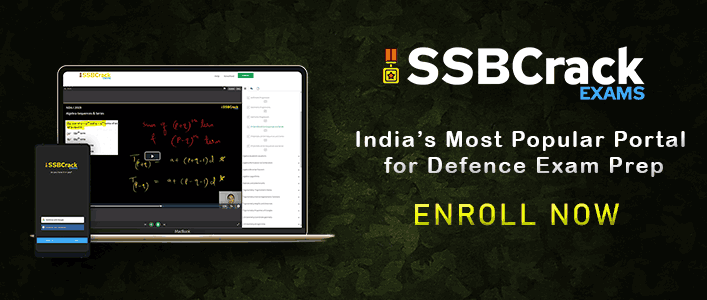# NDA 2 2019 Maths Answer Keys [ALL SETS]Candidates can download the NDA 2 2019 Maths answers keys here, the answers are marked by SSBCrack team on the PDF. NDA 2 2019 paper for Mathematics was a perfect blend of difficult and easy questions simultaneously, thus maintaining the paper difficulty level as “Average”. As usual pattern of this paper, Algebra consisted of 40 questions, Analytical geometry consisted of 12 questions, Statistics and Probability consisted of 20 questions, approximately. Statistics required the conceptual knowledge more this time. Trigonometry, however consisted of 15 questions with a few questions based on ‘periodicity’ as well, which is a bit different from the trends followed in previous years. Commenting on Matrices and Determinants, the weightage was lower than expected, consisting of just 5 questions. Though Vector Algebra was also consisting just a few questions, but the questions require thorough knowledge on concepts of vectors as well as 3-D and 2-D figures together. Integral calculus and Differential Calculus followed approximately 20 questions together, but the pattern was a bit different from previous years. Perhaps, applications of derivatives maintained its importance.

So, basically the students who have prepared well for Algebra, Trigonometry, Differential Calculus and Integral Calculus were in advantage. However, to attempt Matrices and Determinants, Statistics and Probability and Vectors, one should have been conceptually strong. In particular, after analyzing this paper, we can conclude that NDA is expecting more knowledge of ‘Functions’ from students, as most of the questions were based on functions in some or the other ways. This is a very good sign toward the future of Mathematics as this will improve the thinking ability of a student. Also, the topics from Algebra such as Arithmetic Progression and Geometric Progression, Permutation and Combinations, Quadratic equations holds an important place in this examination.

#### NDA 2 2019 Maths Answer Keys [ALL SETS]Download eBooks TAT eBook SRT eBook WAT eBook OIR Test NDA Solved Papers AFCAT Solved Papers Current Affairs eBook 101 Maths Shortcuts